Fillet Weld Under Torsional Loading Calculator Enter value and click on calculate. Result will be displayed.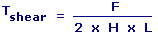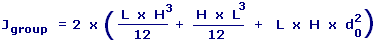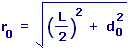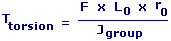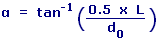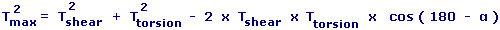F = Applied Force L = Length Of Weld H = Throat Depth Of Weld Tshear = Shear Stress In Weld Due To Shear Force d0 = Distance From Centroid Of Weld Group To Centerline Of Weld L0 = Distance From Centroid Of Weld Group To Applied Force Jgroup = Polar Moment Of Inertia r0 = Radial Distance To Farthest Point On Weld Ttorsion = Shear Stress In Weld Due Torsion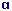= Angle Enclosed Tmax = Maximum Shear Stress in Weld
 Enter your values: Length Of Weld (L): Cm Throat Depth Of Weld (D): Cm Applied Force (F): N Distance From Centroid Of Weld Group To Applied Force (L0): Cm Distance From Centroid Of Weld Group To Centerline Of Weld (d0): Cm Results: Shear Stress In Weld Due To Shear Force: 106 N / m2 Polar Moment Of Inertia: 10-6 N / m4 Shear Stress In Weld Due Torsion: 106 N / m2 Angle Enclosed: ° Maximum Shear Stress In Weld: 106 N / m2

 Web www.calculatoredge.com
 The fillet weld is used to make lap joints, corner joints, and T joints. Fillet weld is roughly triangular in cross-section, although its shape is not always a right triangle or an isosceles triangle. Weld metal is deposited in a corner formed by the fit-up of the two members and penetrates and fuses with the base metal to form the joint.

This calculator is used to calculate the resulting stresses acting in the weld.

A weld of approximately triangular cross section joining two surfaces, approximately at right angles to each other, as in a lap joint.

Stress is a measure of the average amount of force exerted per unit area. It is a measure of the intensity of the total internal forces acting within a body across imaginary internal surfaces, as a reaction to external applied forces and body forces.

Shear stress is a stress state where the stress is parallel or tangential to a face of the material, as opposed to normal stress when the stress is perpendicular to the face.

Polar moment of inertia is a quantity used to predict an object's ability to resist torsion, in objects (or segments of objects) with an invariant circular cross-section and no significant warping or out-of-plane deformation. It is used to calculate the angular displacement of an object subjected to a torque. It is analogous to the area moment of inertia, which characterizes an object's ability to resist bending and is required to calculate displacement.LINKSDISCLAIMERCONTACT US